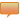Subject Area Lessons

Comprehensive Smartboard Fraction Lessons Unit, 118 Slides!
Posted by Anna Rodriguez on 5/05/11This high quality, professional-looking comprehensive unit
includes over 100 slides of Interactive lessons including
benchmark fractions, equivalent fractions, estimation of
fractions on a number line, comparing fractions, more/less
than 1/2, greater than/less than, visualizing fractions with
like numerators/different denominators, identifying a
fraction of a set, simplifying/reducing a fraction, improper
fractions, mixed numbers, adding and subtracting fractions
and fraction word problems. I have spent months working on
this unit. I taught and revised lessons and spent over 40
hours working on this. I would have jumped at the chance to
save my time by paying \$10.00.